Search

About 58 Search Results Matching Types of Worksheet, Worksheet Section, Generator, Generator Section, Grades matching 5th Grade, Similar to Spelling Worksheets for Words with -oi and -oy Pattern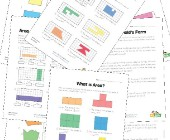Area and Perimeter Worksheets

Help kids learn to calculate the area and perimete...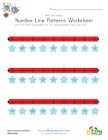Patriotic Number Line Worksheet with Decimals

Fill in the missing numbers on each of the number ...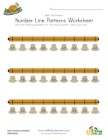Thanksgiving Number Line Worksheet with Decimals

Fill in the missing numbers on each of the number ...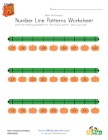Halloween Number Line Worksheet with Decimals

Fill in the missing numbers on each of the number ...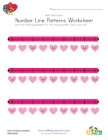Valentine's Day Number Line Worksheet with Decimals

Fill in the missing numbers on each of the number ...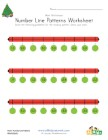Christmas Number Line Worksheet with Decimals

Fill in the missing numbers on each of the number ...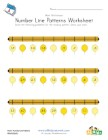New Year Number Line Worksheet with Decimals

Fill in the missing numbers on each of the number ...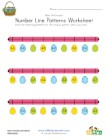Easter Number Line Worksheet with Decimals

Fill in the missing numbers on each of the number ...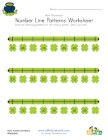St. Patrick's Day Number Line Worksheet with Decimals

Fill in the missing numbers on each of the number ...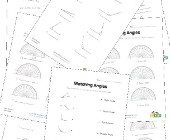Angles Worksheets

Help kids learn about angles with this collection ...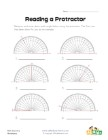Reading a Protractor Worksheet 2

Read each protractor and write down the angles the...